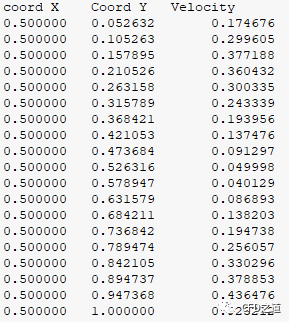Fluent 中提供了 Report 及 Monitor 工具用于监测指定位置的物理量。但如果位置坐标不是固定的，则难以采用监测的方式实现此功能（其实也不是不可以，只不过需要使用 Scheme 计算监测点的坐标），此时可以利用 UDF 将指定坐标点的物理量输出到文件。

# 1 宏介绍

FLUENT_EXPORT CX_Cell_Id *CX_Find_Cell_With_Point(ND_Search *, double v, double time);
• 返回类型为 CX_Cell_ID 的值，之后可以利用 RP_CELL 宏与 RP_THREAD 宏获取到 ct 与 t。
• 参数 ND_search 为一个结构体，其为 Fluent 传递进来的参数，在使用时只需要声明即可。
typedef struct nd_search_struct
{
ND_Table *table;
ND_Table_Dump *table_dump;
int table_num_remain;
ND_Point *points;
double *min;
double *max;
int dim;
int num;
double *values;
void *data;
int total_dump_alloced;
} ND_Search;
• double v：为指定的点坐标数组
• double time：通常指定为 0

FLUENT_EXPORT ND_Search *CX_Start_ND_Point_Search(ND_Search *, cxboolean do_domain, int t_id);
FLUENT_EXPORT ND_Search *CX_End_ND_Point_Search(ND_Search *);

# 2 测试模型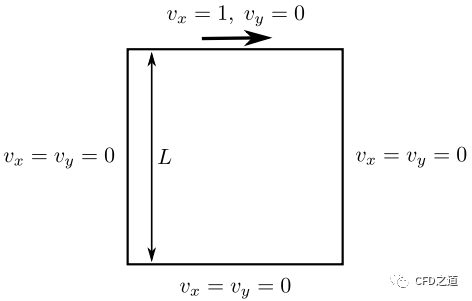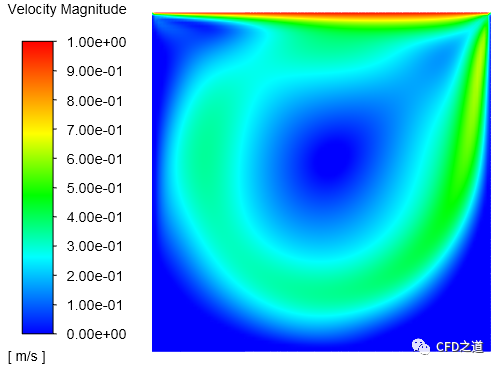• 如下图所示在区域内取一条中轴线，后面用于数据比较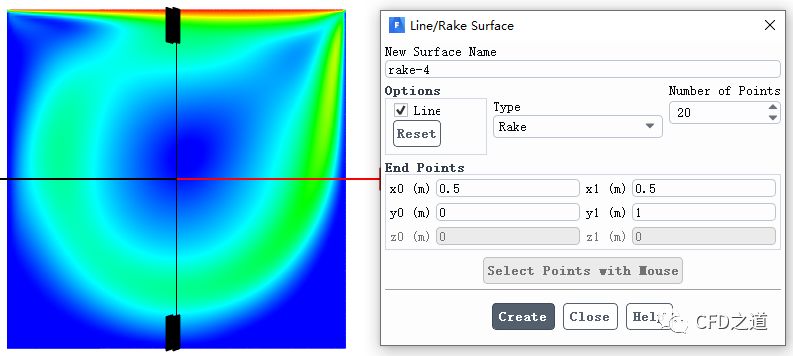• 中轴线上的速度分布如下图所示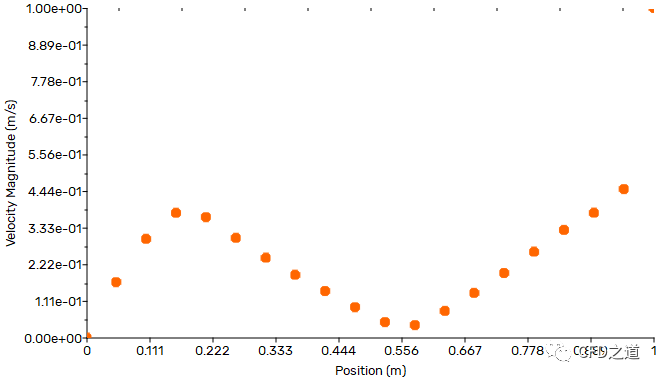# 3 UDF 编写及加载

#include "udf.h"
#include "cxndsearch.h"
static ND_Search *domain_table = NULL;

DEFINE_ON_DEMAND(find_cell)
{
#if !RP_HOST
cell_t c;
CX_Cell_Id *cx_cell;
real velocity;

real P;
real P_Cell;

domain_table = CX_Start_ND_Point_Search(domain_table,TRUE,-1);
Message("coord X\t Coord Y \t Velocity\n");

for(int i =0; i< 20; i++)
{
P = 0.5;
P = i/19.0;

cx_cell = CX_Find_Cell_With_Point(domain_table,P,0.0);
if(cx_cell)
{
c = RP_CELL(cx_cell);
C_CENTROID(P_Cell,c,t);/*可以得到网格中心坐标，本案例并未使用。*/
/*没有直接获取合速度的宏，这里用速度分量自己合成*/
velocity = sqrt(C_U(c,t)*C_U(c,t)+ C_V(c,t)*C_V(c,t));
Message("%f \t %f \t %f\n",P,P,velocity);
}else
{
Message("Could not find cell at [%g,%g]!\n",P,P);
}
}
domain_table = CX_End_ND_Point_Search(domain_table);
#endif
}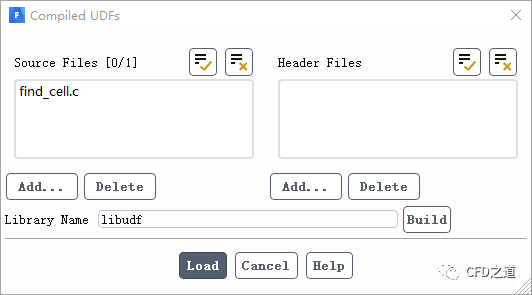• 利用 Execute on Demand 执行 UDF，如下图所示coord X     Coord Y      Velocity
0.500000      0.000000      0.019517
0.500000      0.052632      0.174676
0.500000      0.105263      0.299605
0.500000      0.157895      0.377188
0.500000      0.210526      0.360432
0.500000      0.263158      0.300335
0.500000      0.315789      0.243339
0.500000      0.368421      0.193956
0.500000      0.421053      0.137476
0.500000      0.473684      0.091297
0.500000      0.526316      0.049998
0.500000      0.578947      0.040129
0.500000      0.631579      0.086893
0.500000      0.684211      0.138203
0.500000      0.736842      0.194738
0.500000      0.789474      0.256057
0.500000      0.842105      0.330296
0.500000      0.894737      0.378853
0.500000      0.947368      0.436476
0.500000      1.000000      0.923212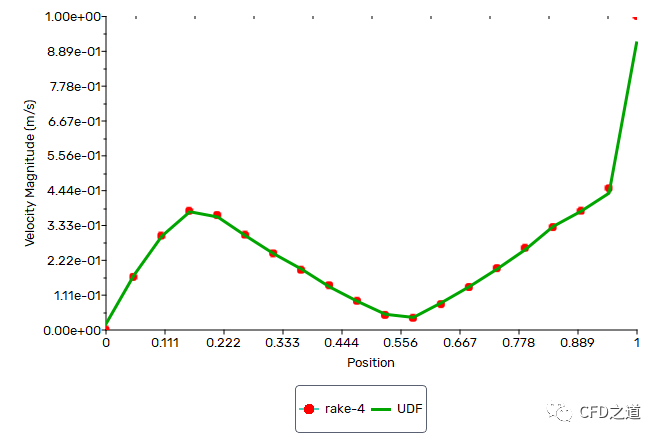# 4 另一种方法

#include "udf.h"
#include "dpm.h"
DEFINE_ON_DEMAND(find_cell)
{
#if !RP_HOST
cell_t c;
CX_Cell_Id cx_cell;
real velocity;
real P;
real P_Cell;

DPM_Init_Oct_Tree_Search();
Message("coord X\t Coord Y \t Velocity\n");
/*若想要输出（0.5,0）点的数据，那就设置i=0*/
for(int i =1; i< 20; i++)
{
P = 0.5;
P = (real)(i/19.0);
cx_cell.ct.c = 0;
cx_cell.ct.t = NULL;
DPM_Locate_Point(P,&cx_cell,0.0,0);

if(NNULLP(cx_cell.ct.t))
{
c = RP_CELL(&cx_cell);
C_CENTROID(P_Cell,c,t);/*可以得到网格中心坐标，本案例没有使用*/
velocity = sqrt(C_U(c,t)*C_U(c,t)+ C_V(c,t)*C_V(c,t));
Message("%f \t %f \t %f \n",P,P,velocity);
}else
{
Message("Could not find cell at [%f,%f]!\n",P,P);
}

}
DPM_End_Oct_Tree_Search();
#endif
}